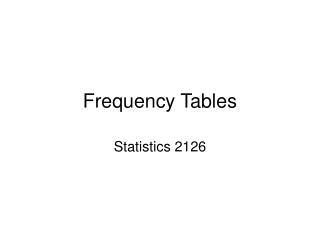Download PresentationFrequency Tables

# Frequency Tables

Télécharger la présentation## Frequency Tables

- - - - - - - - - - - - - - - - - - - - - - - - - - - E N D - - - - - - - - - - - - - - - - - - - - - - - - - - -
##### Presentation Transcript

1. Frequency Tables Statistics 2126

2. Introduction • Say we have a question like “Where are you from?” or “What is your favourite hockey team?” • How would we find out if we have some sort of effect • How would the population be distributed? • We have to somehow come up with a ‘chance’ distribution

3. Say oh rolling a die 60 times

4. How shall we attack this • Observed – expected • 5 -1 0 2 -3 -3 • We can’t just add these up because we would end up with a sum of 0 • Sound familiar? • Square ‘em! • 25 1 0 4 9 9

5. We’re always squaring stuff… • What if the expected values differed? • Well we should weight the squared deviations somehow • Divide by the expected • 25/10+1/10+4/10+9/10+9/10 = 4.8 • Add ‘em all up to get some estimate of the total deviation from the expected

6. Chi squared

7. How to read the χ2 table • df = number of values we can arbitrarily assign • 5 in our case • (there must be 60 rolls in total, so the last number is fixed)

8. Easiest statistic ever… • H0 all the frequencies are the same • Ha they are not • χ2cr = 11.07 • χ2obt < χ2cr • So we do not reject H0

9. For a 2 way table…

10. So… • This is a 3 x 2 table • 3 rows and 2 columns • In general r x c table • We want to test if all of the proportions are equal • H0 p1 = p2 = p3 • Ha all ps are not equal

11. So how do we get the expected values? • We have to compare the observed value to the expected value • But it is hard to get what we would expect by chance • We let the data tell us what to do • (Row total) x (column total) / (table total)

12. Row and Column totals

13. Working out the expecteds • (24 x 24) / 72 for the Yes column • (24 x 48) / 72 for the No column • So we get a table of expected frequencies with 8 in the yes column and 16 in the no column • We expect some relapses but if all the drugs are the same the proportion should be the same

14. Now Do the Test

15. Degrees of Freedom At most we can assign two values arbitrarily

16. So… • In general the degrees of freedom for such a table are (r-1)(c-1) • In our case (3-1)(2-1) • 2cr = 5.99 • We reject H0 • The relapses are distributed differently than chance